# a9.h200c.ch05.solow.growth.pptx

26 de May de 2023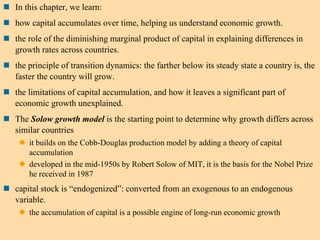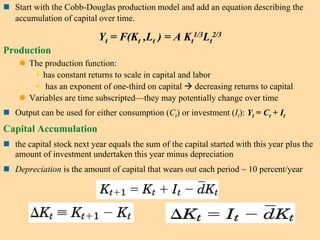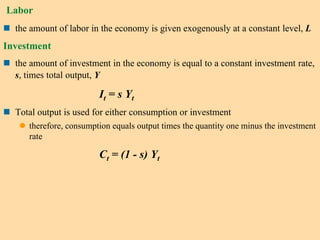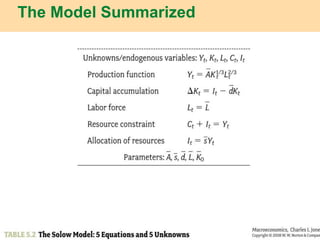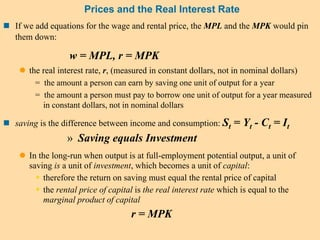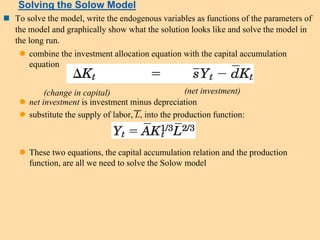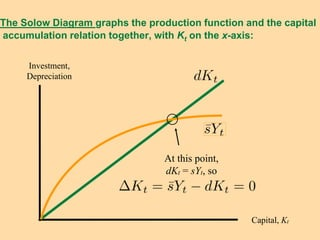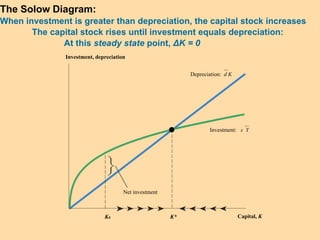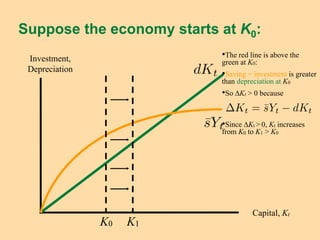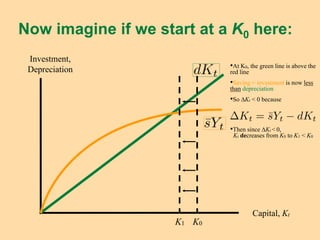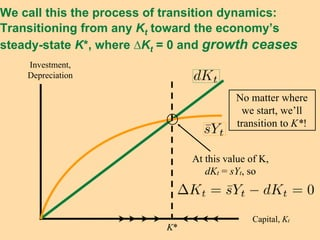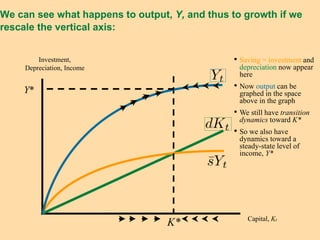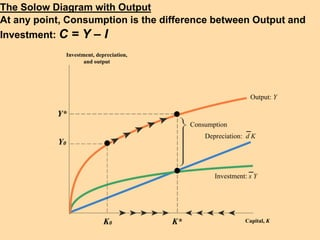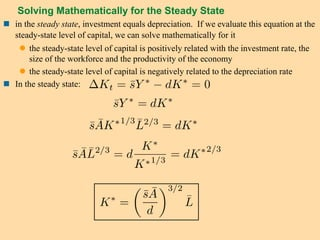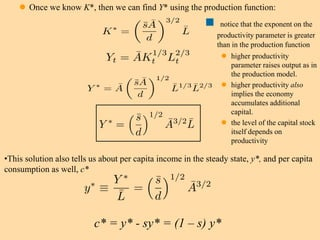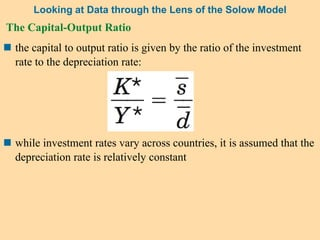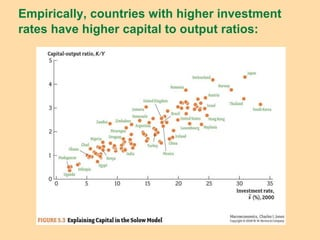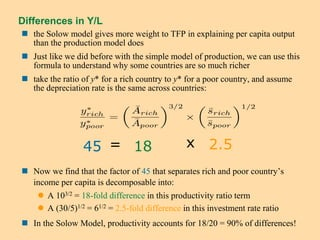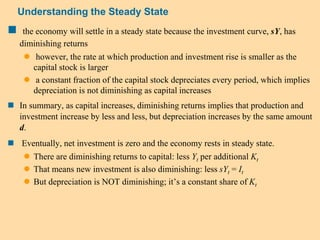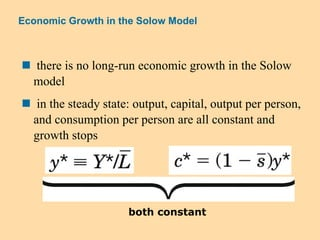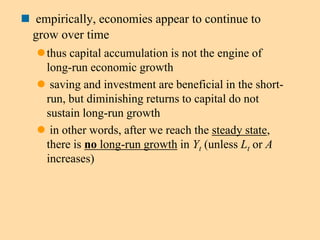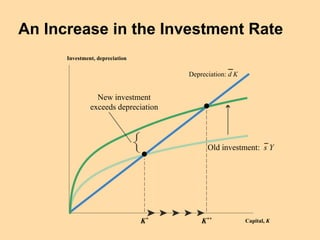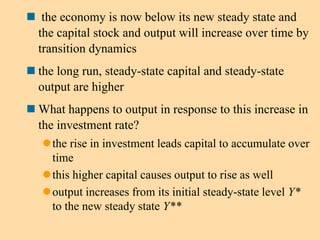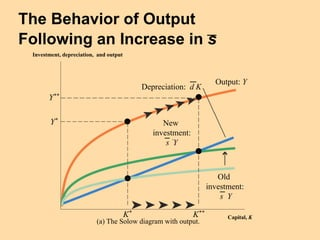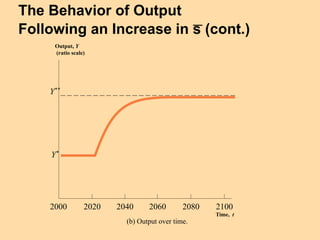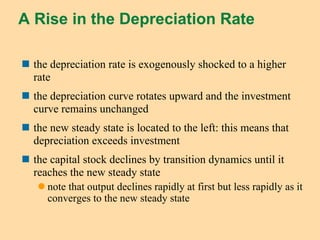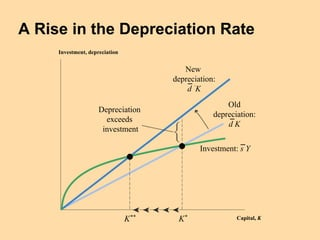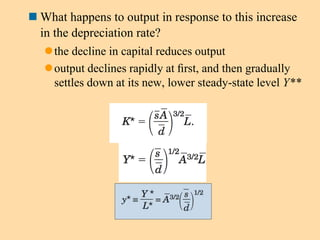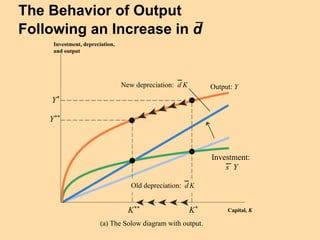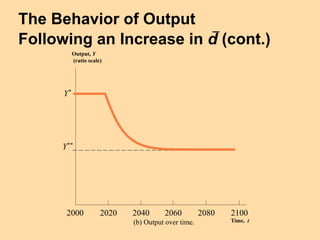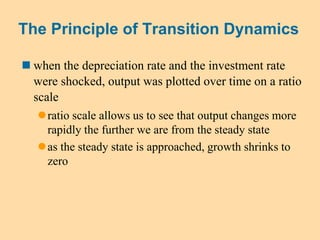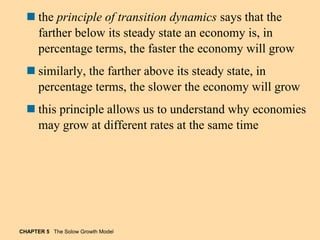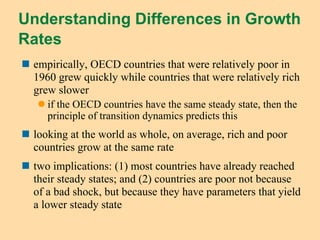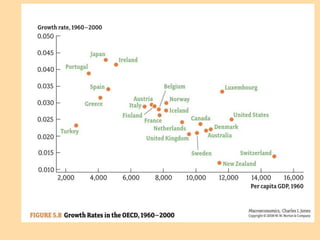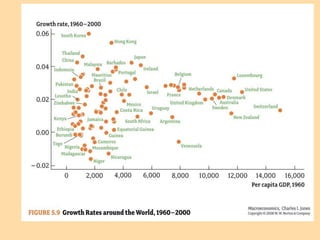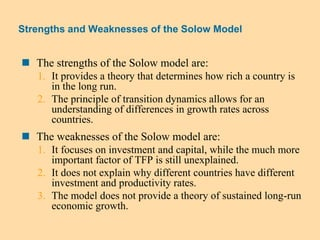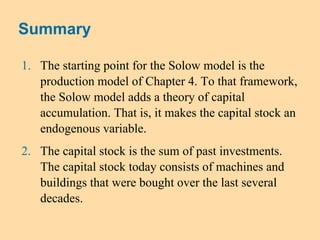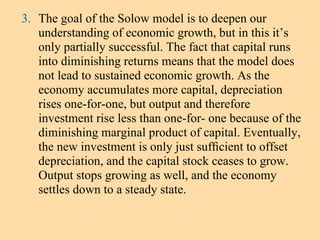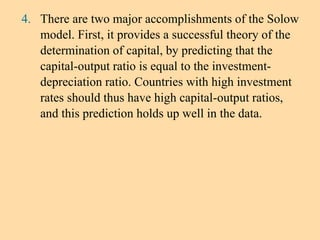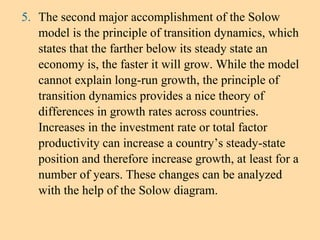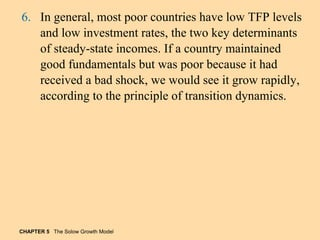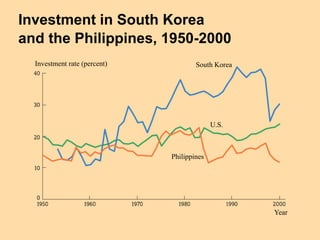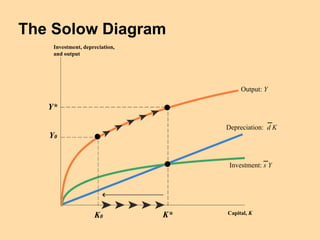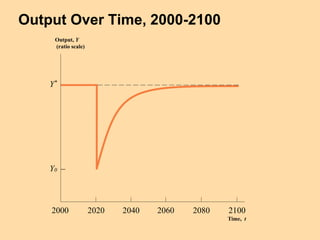1 de 44

### a9.h200c.ch05.solow.growth.pptx

• 1.  In this chapter, we learn:  how capital accumulates over time, helping us understand economic growth.  the role of the diminishing marginal product of capital in explaining differences in growth rates across countries.  the principle of transition dynamics: the farther below its steady state a country is, the faster the country will grow.  the limitations of capital accumulation, and how it leaves a significant part of economic growth unexplained.  The Solow growth model is the starting point to determine why growth differs across similar countries  it builds on the Cobb-Douglas production model by adding a theory of capital accumulation  developed in the mid-1950s by Robert Solow of MIT, it is the basis for the Nobel Prize he received in 1987  capital stock is “endogenized”: converted from an exogenous to an endogenous variable.  the accumulation of capital is a possible engine of long-run economic growth
• 2.  Start with the Cobb-Douglas production model and add an equation describing the accumulation of capital over time. Yt = F(Kt ,Lt ) = A Kt 1/3Lt 2/3 Production  The production function:  has constant returns to scale in capital and labor  has an exponent of one-third on capital  decreasing returns to capital  Variables are time subscripted—they may potentially change over time  Output can be used for either consumption (Ct) or investment (It): Yt = Ct + It Capital Accumulation  the capital stock next year equals the sum of the capital started with this year plus the amount of investment undertaken this year minus depreciation  Depreciation is the amount of capital that wears out each period ~ 10 percent/year
• 3. Labor  the amount of labor in the economy is given exogenously at a constant level, L Investment  the amount of investment in the economy is equal to a constant investment rate, s, times total output, Y It = s Yt  Total output is used for either consumption or investment  therefore, consumption equals output times the quantity one minus the investment rate Ct = (1 - s) Yt
• 5. Prices and the Real Interest Rate  If we add equations for the wage and rental price, the MPL and the MPK would pin them down: w = MPL, r = MPK  the real interest rate, r, (measured in constant dollars, not in nominal dollars) = the amount a person can earn by saving one unit of output for a year = the amount a person must pay to borrow one unit of output for a year measured in constant dollars, not in nominal dollars  saving is the difference between income and consumption: St = Yt - Ct = It » Saving equals Investment  In the long-run when output is at full-employment potential output, a unit of saving is a unit of investment, which becomes a unit of capital:  therefore the return on saving must equal the rental price of capital  the rental price of capital is the real interest rate which is equal to the marginal product of capital r = MPK
• 6.  To solve the model, write the endogenous variables as functions of the parameters of the model and graphically show what the solution looks like and solve the model in the long run.  combine the investment allocation equation with the capital accumulation equation  net investment is investment minus depreciation  substitute the supply of labor, L, into the production function:  These two equations, the capital accumulation relation and the production function, are all we need to solve the Solow model (change in capital) (net investment) Solving the Solow Model
• 7. Investment, Depreciation Capital, Kt At this point, dKt = sYt, so The Solow Diagram graphs the production function and the capital accumulation relation together, with Kt on the x-axis:
• 8. The Solow Diagram: When investment is greater than depreciation, the capital stock increases The capital stock rises until investment equals depreciation: At this steady state point, ΔK = 0 Depreciation: d K Investment: s Y Investment, depreciation Capital, K K* Net investment K0
• 9. Suppose the economy starts at K0: Investment, Depreciation Capital, Kt K0 •The red line is above the green at K0: •Saving = investment is greater than depreciation at K0 •So ∆Kt > 0 because •Since ∆Kt > 0, Kt increases from K0 to K1 > K0 K1
• 10. Now imagine if we start at a K0 here: Investment, Depreciation Capital, Kt K0 •At K0, the green line is above the red line •Saving = investment is now less than depreciation •So ∆Kt < 0 because •Then since ∆Kt < 0, Kt decreases from K0 to K1 < K0 K1
• 11. We call this the process of transition dynamics: Transitioning from any Kt toward the economy’s steady-state K*, where ∆Kt = 0 and growth ceases Investment, Depreciation Capital, Kt At this value of K, dKt = sYt, so K* No matter where we start, we’ll transition to K*!
• 12. We can see what happens to output, Y, and thus to growth if we rescale the vertical axis: Investment, Depreciation, Income Capital, Kt K* • Saving = investment and depreciation now appear here • Now output can be graphed in the space above in the graph • We still have transition dynamics toward K* • So we also have dynamics toward a steady-state level of income, Y* Y*
• 13. The Solow Diagram with Output At any point, Consumption is the difference between Output and Investment: C = Y – I Investment, depreciation, and output Capital, K Y0 K0 Y* K* Consumption Depreciation: d K Investment: s Y Output: Y
• 14.  in the steady state, investment equals depreciation. If we evaluate this equation at the steady-state level of capital, we can solve mathematically for it  the steady-state level of capital is positively related with the investment rate, the size of the workforce and the productivity of the economy  the steady-state level of capital is negatively related to the depreciation rate Solving Mathematically for the Steady State  In the steady state:
• 15.  Once we know K*, then we can find Y* using the production function: •This solution also tells us about per capita income in the steady state, y*, and per capita consumption as well, c* c* = y* - sy* = (1 – s) y*  notice that the exponent on the productivity parameter is greater than in the production function  higher productivity parameter raises output as in the production model.  higher productivity also implies the economy accumulates additional capital.  the level of the capital stock itself depends on productivity
• 16. The Capital-Output Ratio  the capital to output ratio is given by the ratio of the investment rate to the depreciation rate:  while investment rates vary across countries, it is assumed that the depreciation rate is relatively constant Looking at Data through the Lens of the Solow Model
• 17. Empirically, countries with higher investment rates have higher capital to output ratios:
• 18. Differences in Y/L  the Solow model gives more weight to TFP in explaining per capita output than the production model does  Just like we did before with the simple model of production, we can use this formula to understand why some countries are so much richer  take the ratio of y* for a rich country to y* for a poor country, and assume the depreciation rate is the same across countries:  Now we find that the factor of 45 that separates rich and poor country’s income per capita is decomposable into:  A 103/2 = 18-fold difference in this productivity ratio term  A (30/5)1/2 = 61/2 = 2.5-fold difference in this investment rate ratio  In the Solow Model, productivity accounts for 18/20 = 90% of differences! 45 = 18 x 2.5
• 19.  the economy will settle in a steady state because the investment curve, sY, has diminishing returns  however, the rate at which production and investment rise is smaller as the capital stock is larger  a constant fraction of the capital stock depreciates every period, which implies depreciation is not diminishing as capital increases  In summary, as capital increases, diminishing returns implies that production and investment increase by less and less, but depreciation increases by the same amount d.  Eventually, net investment is zero and the economy rests in steady state.  There are diminishing returns to capital: less Yt per additional Kt  That means new investment is also diminishing: less sYt = It  But depreciation is NOT diminishing; it’s a constant share of Kt Understanding the Steady State
• 20.  there is no long-run economic growth in the Solow model  in the steady state: output, capital, output per person, and consumption per person are all constant and growth stops both constant Economic Growth in the Solow Model
• 21.  empirically, economies appear to continue to grow over time thus capital accumulation is not the engine of long-run economic growth  saving and investment are beneficial in the short- run, but diminishing returns to capital do not sustain long-run growth  in other words, after we reach the steady state, there is no long-run growth in Yt (unless Lt or A increases)
• 22. Investment, depreciation Capital, K New investment exceeds depreciation Depreciation: d K K** K* Old investment: s Y An Increase in the Investment Rate
• 23.  the economy is now below its new steady state and the capital stock and output will increase over time by transition dynamics  the long run, steady-state capital and steady-state output are higher  What happens to output in response to this increase in the investment rate? the rise in investment leads capital to accumulate over time this higher capital causes output to rise as well output increases from its initial steady-state level Y* to the new steady state Y**
• 24. The Behavior of Output Following an Increase in s Investment, depreciation, and output Capital, K Y* K* Y** K** Depreciation: d K Output: Y New investment: s ‘Y Old investment: s Y (a) The Solow diagram with output.
• 25. The Behavior of Output Following an Increase in s (cont.) (b) Output over time. Y* Y** Output, Y (ratio scale) Time, t 2000 2020 2040 2060 2080 2100
• 26. A Rise in the Depreciation Rate  the depreciation rate is exogenously shocked to a higher rate  the depreciation curve rotates upward and the investment curve remains unchanged  the new steady state is located to the left: this means that depreciation exceeds investment  the capital stock declines by transition dynamics until it reaches the new steady state  note that output declines rapidly at first but less rapidly as it converges to the new steady state
• 27. A Rise in the Depreciation Rate Investment, depreciation Capital, K Depreciation exceeds investment K** K* New depreciation: d ‘K Old depreciation: d K Investment: s Y
• 28.  What happens to output in response to this increase in the depreciation rate? the decline in capital reduces output output declines rapidly at ﬁrst, and then gradually settles down at its new, lower steady-state level Y**
• 29. The Behavior of Output Following an Increase in d Investment, depreciation, and output Capital, K New depreciation: d‘K Output: Y Investment: s Y Old depreciation: d K Y** K** Y* K* (a) The Solow diagram with output.
• 30. The Behavior of Output Following an Increase in d (cont.) Output, Y (ratio scale) Time, t 2000 2020 2040 2060 2080 2100 Y** Y* (b) Output over time.
• 31. The Principle of Transition Dynamics  when the depreciation rate and the investment rate were shocked, output was plotted over time on a ratio scale ratio scale allows us to see that output changes more rapidly the further we are from the steady state as the steady state is approached, growth shrinks to zero
• 32. CHAPTER 5 The Solow Growth Model  the principle of transition dynamics says that the farther below its steady state an economy is, in percentage terms, the faster the economy will grow  similarly, the farther above its steady state, in percentage terms, the slower the economy will grow  this principle allows us to understand why economies may grow at different rates at the same time
• 33. Understanding Differences in Growth Rates  empirically, OECD countries that were relatively poor in 1960 grew quickly while countries that were relatively rich grew slower  if the OECD countries have the same steady state, then the principle of transition dynamics predicts this  looking at the world as whole, on average, rich and poor countries grow at the same rate  two implications: (1) most countries have already reached their steady states; and (2) countries are poor not because of a bad shock, but because they have parameters that yield a lower steady state
• 36. Strengths and Weaknesses of the Solow Model  The strengths of the Solow model are: 1. It provides a theory that determines how rich a country is in the long run. 2. The principle of transition dynamics allows for an understanding of differences in growth rates across countries.  The weaknesses of the Solow model are: 1. It focuses on investment and capital, while the much more important factor of TFP is still unexplained. 2. It does not explain why different countries have different investment and productivity rates. 3. The model does not provide a theory of sustained long-run economic growth.
• 37. Summary 1. The starting point for the Solow model is the production model of Chapter 4. To that framework, the Solow model adds a theory of capital accumulation. That is, it makes the capital stock an endogenous variable. 2. The capital stock is the sum of past investments. The capital stock today consists of machines and buildings that were bought over the last several decades.
• 38. 3. The goal of the Solow model is to deepen our understanding of economic growth, but in this it’s only partially successful. The fact that capital runs into diminishing returns means that the model does not lead to sustained economic growth. As the economy accumulates more capital, depreciation rises one-for-one, but output and therefore investment rise less than one-for- one because of the diminishing marginal product of capital. Eventually, the new investment is only just sufﬁcient to offset depreciation, and the capital stock ceases to grow. Output stops growing as well, and the economy settles down to a steady state.
• 39. 4. There are two major accomplishments of the Solow model. First, it provides a successful theory of the determination of capital, by predicting that the capital-output ratio is equal to the investment- depreciation ratio. Countries with high investment rates should thus have high capital-output ratios, and this prediction holds up well in the data.
• 40. 5. The second major accomplishment of the Solow model is the principle of transition dynamics, which states that the farther below its steady state an economy is, the faster it will grow. While the model cannot explain long-run growth, the principle of transition dynamics provides a nice theory of differences in growth rates across countries. Increases in the investment rate or total factor productivity can increase a country’s steady-state position and therefore increase growth, at least for a number of years. These changes can be analyzed with the help of the Solow diagram.
• 41. CHAPTER 5 The Solow Growth Model 6. In general, most poor countries have low TFP levels and low investment rates, the two key determinants of steady-state incomes. If a country maintained good fundamentals but was poor because it had received a bad shock, we would see it grow rapidly, according to the principle of transition dynamics.
• 42. Investment in South Korea and the Philippines, 1950-2000 Investment rate (percent) Year U.S. South Korea Philippines
• 43. The Solow Diagram Investment, depreciation, and output Capital, K Y0 K0 Y* K* Output: Y Depreciation: d K Investment: s Y
• 44. Output Over Time, 2000-2100 Output, Y (ratio scale) Time, t 2000 2020 2040 2060 2080 2100 Y* Y0